• Topic Specification## Sequences and Nth Term Revision

Try it yourself.

• Example Questions
• Worksheets 1## Filter by Level

Filter by exam board, sequences and nth term.

In maths, a sequence is a list of numbers, algebraic terms, shapes, or other mathematical objects that follow a pattern or rule. There are two different ways you will be expected to work out a sequence:

• A term-to-term rule – each term in the sequence is calculated by performing a fixed set of operations (such as “multiply by 2 and add 3 ”) to the term(s) before it.
• A position-to-term rule – each term in the sequence is calculated according to its position in the sequence. Usually, this takes the form of an n^{th} term formula.

## Finding a Sequence from the n^{th} Term

Some sequences have a rule called an n^{th} term rule , which tells you how to generate terms.

The n^{th} term rule is always an expression in n .

To find the first term in the sequence , substitute n=1 into the expression . To find the second term in the sequence , substitute n=2 into the expression . And so on.

Example: A sequence has the n^{th} term rule 2n+1 . Find the first four terms .

First term: n=1

Second term: n=2

Third term: n=3

Fourth term: n=4

The first four terms are: 3 , 5 , 7 and 9 .

## Finding the n^{th} term for a Linear Sequence

Linear sequences (or arithmetic progressions) are sequences that increase or decrease by the same amount between each term.

Want a way to express any term in a concise mathematical way? This can be done using the n^{th} term formula. This is a rule that gives you the value of any term in the sequence in the form,

Where a and b are numbers to be determined.

Example: Find the n^{th} term for the following sequence,    3, \, 7, \,11, \,15, \,19, \, ...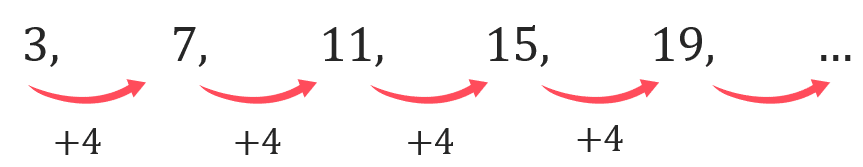Step 1: Find the Common Difference ( a )

The  common difference  is the amount the sequence increases (or decreases) each time.

a=4 , because a is always the difference between each term.

Step 2: Determine if you need to Add or Subtract anything ( b )

To work out b , consider the sequence formed by putting n=1, 2, 3, 4, 5 into 4n :

4, 8, 12, 16, 20

What’s the difference between these terms and our actual sequence? They’re all too big by 1 . So, to make our original sequence, we must subtract 1 from 4n .

Step 3: Write the formula in the correct form ( an+b )

Thus, our n^{th} term formula is

## Geometric Sequences

Another type of sequence is a geometric sequence or geometric progression.

In a geometric sequence , you multiply each term by a common ratio to get to the next term. For example,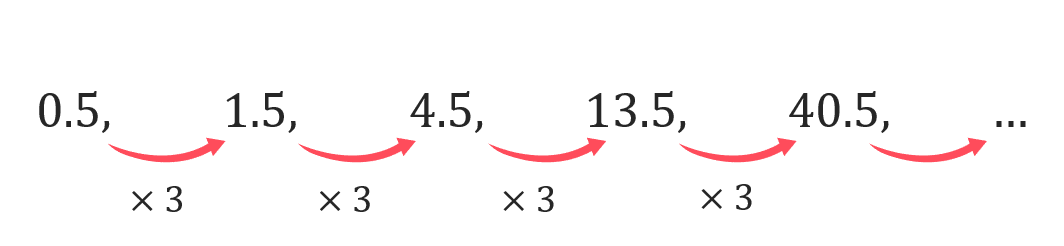is a geometric progression where to get get to the next term you have to multiply the previous term by the common ratio. Therefore to find the next two terms of this sequence we have to multiply the preceding term by 3 , so

40.5\times 3=121.5 and  121.5\times 3 =364.5

## Geometric Sequences involving Surds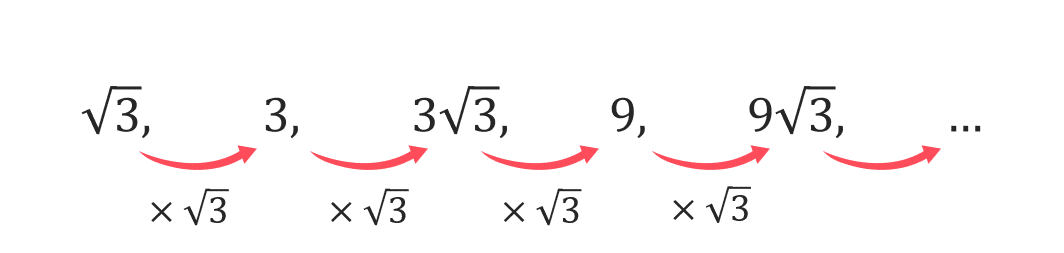is a geometric progression where the common ratio is not a rational number. The method of finding the next two terms of this sequence is the same as before, multiply the preceding term by \sqrt{3} , so

9\sqrt{3}\times 3=27 and  27\times \sqrt{3} = 27\sqrt{3}

## Other Types of Sequences

There are other types of sequences you should be familiar with:

## Triangular Numbers

Triangular numbers are numbers that can be represented as an equilateral triangle of dots.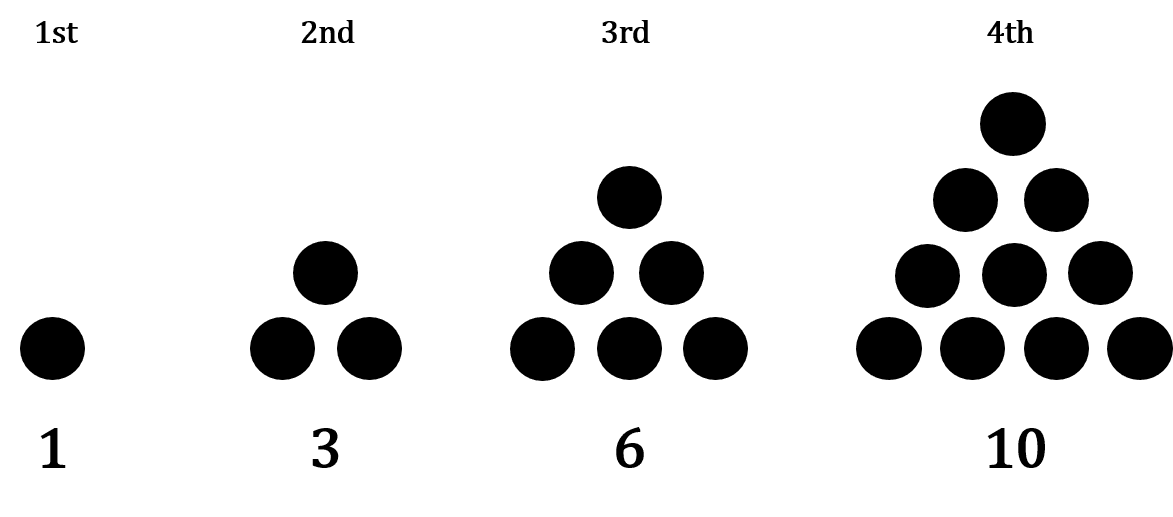The n^{th} term is \dfrac{n(n+1)}{2} , giving

1, 3, 6, 10, 15, 21, ...

## Square Numbers

These sequences are made up of square numbers so the n^{th} term is n^2 , giving

1, 4, 9, 16, 25, 36, 49, ...

## Cubic Numbers

These sequences are made up of cubic numbers so the n^{th} term is n^3 , giving

1, 8, 27, 64, 125, 216, ...

## The Fibonacci Numbers

The first few terms of the Fibonacci sequence are:

1, 1, 2, 3, 5, 8, 13, 21, ...

This is a famous sequence that you need to recognise. The rule is the previous 2 terms are added together in the sequence to get the next term.

Subscript notation can be used to denote position to term and term to term rules.

For example

x_{n+1}=3x_n-4 shows that you multiply the previous term by 3 and subtract 4 to get the next term.

y_n=5n+3 gives you a rule for the n th term of a sequence.

## Example 1: Linear Sequences

Find the n^{th} term formula for the sequence -2, 5, 12, 19, 26 .

The first step is to find the common difference between each term.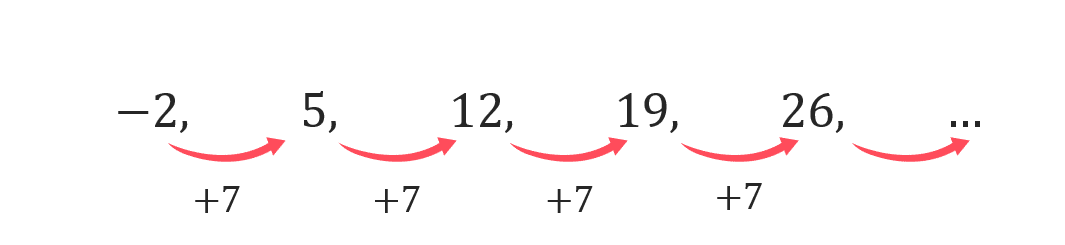Hence we can write the n^{th} term as,

To work out b , consider the sequence formed by putting n=1, 2, 3, 4, 5 into u_n=7n :

7, 14, 21, 28, 35

The difference between these numbers and our sequence is we need to subtract 9 from each term. Thus, our n^{th} term is

## Example 2: Determining if a Value is Part of a Sequence

The first five terms of a sequence are:

-3, 1, 5, 9, 13

Determine if 1143 is part of this sequence.Step 1: First we must find the n^{th} term of the sequence of the sequence as before, this gives

Step 2:  Next we need to write the n^{th} term as an euqation equal to 1143 and solve for n

If n solves to give an integer, then 1143 is part of the sequence.

\begin{aligned} 4n-7&=1143 \\ 4n & = 1150 \\ n&= 287.5\end{aligned}

As 287.5 is not an integer, 1143 must not be part of the sequence.

## Example 3: Solving Problems involving Sequences

The sum of two consecutive terms in a sequence given by the n^{th} term, 3n+8 is 109 . Find the values of these two terms.

In this case we have to first set up an equation, setting the first term as n and the second as n+1 , such that,

\begin{aligned} 3n+8+3(n+1)+8 & = 109 \\ 3n + 8 + 3n + 3 + 8 & = 109 \\ 6n +19 &= 109 \\ 6n &= 90 \\ n &=15 \end{aligned}

Hence, it is the 15 th and 16 th terms we are looking for which are,

3(15)+8 = 53 and 3(16) +8 = 56

## Sequences and Nth Term Example Questions

Question 1: A sequence has the n^{th} term 4n+1

a) Find the 12^{th} term in this sequence

b) A term in this sequence is 77 . Find the position of this term in the sequence.

a) To find the 12^{th}  term of this sequence, we will substitute n=12 into the formula given.

So, the 12^{th} term is 49

b) Every term in this sequence is generated when an integer value of n is substituted into 4n+1

Hence if we set 77 to equal 4n+1 , we can determine its position in the sequence,

making n the subject by subtracting 1 then dividing by 4 ,

n=\dfrac{77-1}{4}=19

Hence 77 is the 19^{th} term in the sequence.Gold Standard Education

Question 2: A sequence is defined by the formula 5n-4

a) Work out the first 5 terms of this sequence.

b) Explain why 108 is not a term in this sequence.

a) To generate the first 5 terms of this sequence, we will substitute n=1, 2, 3, 4, 5 into the formula given.

\begin{aligned}1 &=5(1)-4 =1 \\ 2 &=5(2)-4 =6 \\ 3 & =5(3)-4=11 \\ 4 &=5(4)-4=16 \\ 5 &=5(5)-4=21 \end{aligned}

So, the first 5 terms are 1 , 6 , 11 , 16 , and 21

b) Every term in this sequence is generated when an integer value of n is substituted into 5n-4 .

If we set 108 to equal 5n-4 , we can determine if it is a part of the sequence or not. If the value of n is a whole number then it is part of the sequence, hence

making n the subject by adding 4 then dividing by 5 ,

n=\dfrac{112}{5}=22.4

As there is no “ 22.4^{th} ” position in the sequence, it must the case that 108 is not a term in this sequence.

Question 3: The first 5 terms of an arithmetic progression are

-3,\,\,2,\,\,7,\,\,12,\,\,17

Find the formula for the n^{th} term of this sequence.

We are told it is an arithmetic progression and so must have n^{th} formula: an+b . To find a , we must inspect the difference between each term which is 5 , hence a=5 .

Then, to find b , let’s consider the sequence generated by 5n :

5,\,\,10,\,\,15,\,\,20,\,\,25

Every term is this sequence is bigger than the corresponding terms in the original sequence by 8 . So, to get to the original sequence, we will have to subtract 8 from every term in this sequence. In other words, the n^{th} term formula for our sequence in question is

## Sequences and Nth Term Worksheet and Example Questions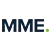## (NEW) Sequences and Nth Term (Linear) Exam Style Questions - MME

Sequences and nth term drill questions, sequences (2) - drill questions, sequences & nth term - drill questions, related topics, quadratic sequences, where next, previous gcse maths topic, algebraic fractions, gcse maths revision home, go back to the main gcse maths topic list, next gcse maths topic.

By clicking continue and using our website you are consenting to our use of cookies in accordance with our Cookie Policy

## Report a Question

You must be logged in to vote for this question..## Corbettmaths

• International
• Schools directory
• Resources Jobs Schools directory News Search## GCSE MATHS sequences worksheet

Subject: Mathematics

Age range: 14-16

Resource type: Worksheet/ActivityLast updated

21 January 2021

• Share through email
• Share through pinterestA GCSE Maths Worksheet covering sequences and the nth term

Suitable for GCSE Students sitting the 9 – 1, A-Level Maths students revision and some Key Stage 3 students.

Suitable for all the big examination boards including AQA, Edexcel, OCR and WJEC.

This was taken from my 150 Page workbook which covers many more maths topics. Please check it out here https://www.tes.com/teaching-resource/gcse-mathematics-150-page-summer-holiday-workbook-11940692

Or alternatively Check my store for other individual free worksheets such as this one. If you like it please leave a comment below, use the rating system or send us a message. All feedback is valued & welcome.

Creative Commons "Sharealike"

It's good to leave some feedback.

Something went wrong, please try again later.

Superb - many thanks :-)

Empty reply does not make any sense for the end user

## KavitaPhillippo

Worksheet was quit useful

Report this resource to let us know if it violates our terms and conditions. Our customer service team will review your report and will be in touch.

## Linear Sequences: Worksheets with Answers

Whether you want a homework, some cover work, or a lovely bit of extra practise, this is the place for you. And best of all they all (well, most!) come with answers.

Mathster is a fantastic resource for creating online and paper-based assessments and homeworks. They have kindly allowed me to create 3 editable versions of each worksheet, complete with answers.

Corbett Maths offers outstanding, original exam style questions on any topic, as well as videos, past papers and 5-a-day. It really is one of the very best websites around.

• Schools (0)

## Lesson Resources

"Quite simply, his lessons and activities are brilliant. They are clearly laid out, contain examples, notes, questions and answers, and cover pretty much everything from key stage 3 right up to further maths A-level. They are all PowerPoint presentations or Word documents, so can be adapted, edited and merged with your existing lessons. Our students and teachers are currently Dr Frost mad!"

Craig Barton, Head of TES Maths Panel

## Resource Collections

• Pearson Edexcel A Level Maths Slides
• Pearson Edexcel A Level FM Slides
• Virtual Calculators

## Revision for UK curriculum

• 'Full Coverage' GCSE
• 'Full Coverage' A Level
• 'Full Coverage' IGCSE FM
• UKMT Database
• Year 12 Zeta Club

## Sequences Worksheet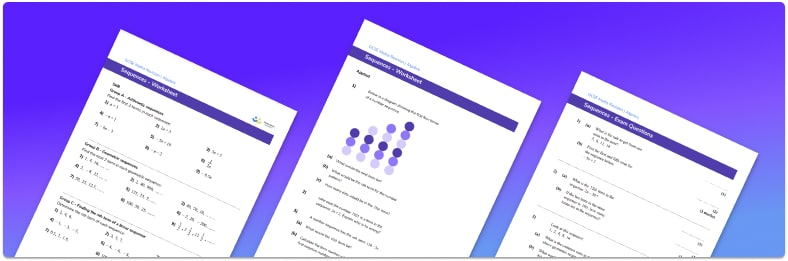• Section 1 of the sequences worksheet contains 20+ skills-based sequences questions, in 3 groups to support differentiation
• Section 2 of the resource contains 3 applied sequences questions with a mix of worded problems and deeper problem solving questions
• Section 3 contains 3 foundation and higher level GCSE practice and exam questions
• Answers and a mark scheme for all sequences questions are provided
• Questions follow variation theory with plenty of opportunities for students to work independently at their own level
• All questions created by fully qualified expert secondary maths teachers
• Suitable for GCSE maths revision for AQA, OCR and Edexcel exam boards

• Job Title * Job title Headteacher Deputy Head Assistant Head Head of Maths Deputy Head of Maths Trust Leader Primary SLT Other SLT Secondary Maths Teacher Primary Teacher Teaching Assistant Tutor Parent Student Other
• Name This field is for validation purposes and should be left unchanged.

You can unsubscribe at any time (each email we send will contain an easy way to unsubscribe). To find out more about how we use your data, see our privacy policy .Raise maths attainment across your school with hundreds of flexible and easy to use GCSE maths worksheets and lessons designed by teachers for teachers.

## Sequences at a glance

Sequences are a set of numbers that have a starting number and go on forever. Number sequences can increase or decrease and can be whole numbers, fractions or decimals.

There are different ways to classify a sequence including arithmetic sequences and geometric sequences. Arithmetic sequences have a common difference and geometric sequences have a common ratio. The common difference or common ratio can be used to continue a sequence, or to find missing terms within the sequence and may be referred to as the term-to-term rule.

Arithmetic sequences are based on multiples which can be used to find the nth term rule (or nth term formula) by calculating the common difference by subtracting two consecutive terms in the sequence. The common difference gives the coefficient of the n in the nth term formula.

The nth term can be referred to as the position-to-term rule and can be used to find a particular term in the sequence or used to check if a particular value is in the sequence or not. A sequence that is increasing or decreasing by the same amount is called a linear sequence.

There are other types of sequences such as the square numbers that form a sequence which is involved in quadratic sequences. Other non-linear sequences include the cube numbers and the powers of 10. There is also the Fibonacci sequence where the next term is found by adding the previous two terms. Triangular numbers is another special sequence which is useful to know.

Looking forward, students can progress with other  sequences worksheets  and on to additional algebra worksheets , for example a simplifying expressions worksheet , straight line graph or simultaneous equations worksheet .For more teaching and learning support on Algebra our GCSE maths lessons provide step by step support for all GCSE maths concepts.

## Related worksheets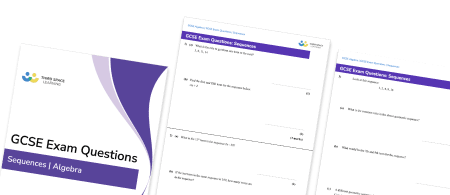## GCSE Exam Questions – Sequences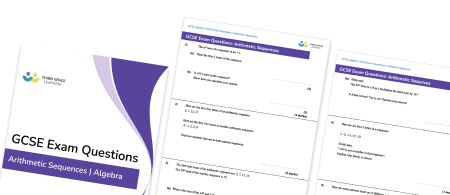## GCSE Exam Questions – Arithmetic Sequences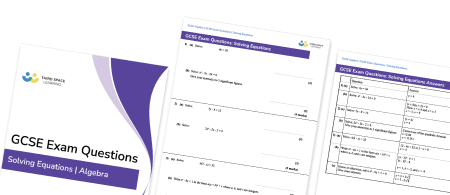## GCSE Exam Questions – Solving Equations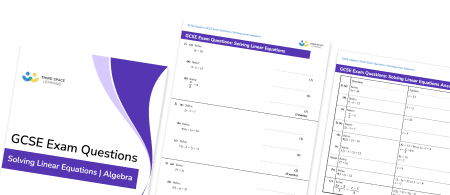## GCSE Exam Questions – Solving Linear Equations

Popular for gcse.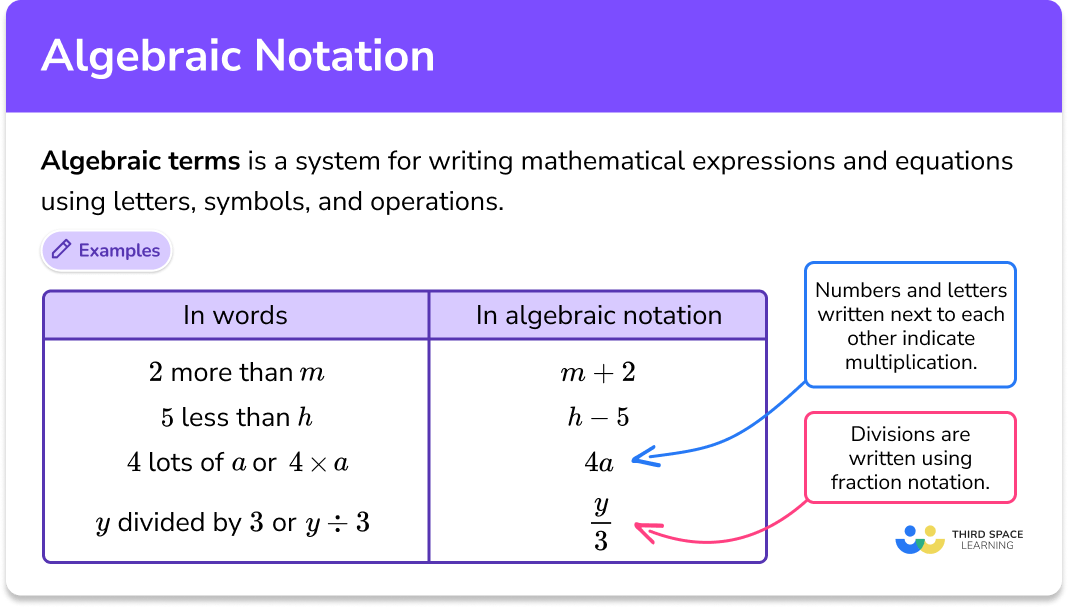## Do you have KS4 students who need more focused attention to succeed at GCSE?There will be students in your class who require individual attention to help them succeed in their maths GCSEs. In a class of 30, it’s not always easy to provide.

Help your students feel confident with exam-style questions and the strategies they’ll need to answer them correctly with our dedicated GCSE maths revision programme.

Lessons are selected to provide support where each student needs it most, and specially-trained GCSE maths tutors adapt the pitch and pace of each lesson. This ensures a personalised revision programme that raises grades and boosts confidence.

## Privacy Overview#### IMAGES2. Sequences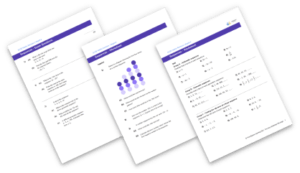3. Sequence Practice Worksheets4. 5 worksheets covering all types of sequences for new GCSE5. GCSE MATHS sequences worksheet6. Sequences GCSE/IGCSE worksheets (with solutions)#### VIDEO

2. GCSE Maths

3. Using Nth Terms and Sequences

4. Nth Term of Linear Sequences

5. Geometric Progressions

6. Geometric Sequences

1. Tips and Tricks for Making the Most of Teachers for Teachers Worksheets

Teachers for Teachers worksheets are a great way to provide students with engaging and educational activities. With the right approach, these worksheets can be used to help students learn and retain information in an effective way. Here are...

2. Make Math Fun with Engaging Math Practice Worksheets

Math can be a challenging subject for many students, but it doesn’t have to be. With the help of engaging math practice worksheets, you can make math fun and help your students develop their math skills. Here are some tips on how to make ma...

3. Where to Find Printable Reading Worksheets

Teaching children to read is an important skill they’ll use for the rest of their lives. When children need extra practice using their reading skills, it helps to have worksheets available. You can find an assortment of printable reading wo...

4. SEQUENCES

Edexcel GCSE. Mathematics (Linear) – 1MA0. SEQUENCES. Materials required for examination. Items included with question papers. Ruler graduated in centimetres

5. GCSE (1

GCSE (1 – 9). Sequences. Name: Instructions. • Use black ink or ball-point pen. • Answer all Questions. • Answer the Questions in

6. Sequences and Finding the nth Term GCSE Maths

7. Sequences nth Term Practice Questions

Corbettmaths. Videos, worksheets, 5-a-day and much more ... GCSE Revision Cards. 5-a-day Workbooks. Primary Study Cards. Search for

8. 10 Sequences

Second differences. 6. 6. 6. (c) Again, the second differences are constant; this time they are all 6. Note. For a sequence defined by a quadratic formula, the

9. Sequences

What is a sequence? A number sequence is a set of numbers that follow a particular pattern or rule to get from term to term. There are four main types of

10. GCSE MATHS sequences worksheet

A GCSE Maths Worksheet covering sequences and the nth term Suitable for GCSE Students sitting the 9 – 1, A-Level Maths students revision and some Key Stage

11. Nth Term Worksheet

If the nth term was then the sequence would be 3, 6, 9, 12, 15, … Step 3: Compare the estimate and work out the difference between the sequences

12. Linear Sequences: Worksheets with Answers

They have kindly allowed me to create 3 editable versions of each worksheet, complete with answers. Worksheet Name, 1, 2, 3. Create Sequence - Given nth Term, 1